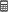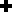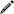# Tips & Tricks - Calculation

This article explains how you can perform applications in applications using the formula editor.A simple view table contains a "Value" column where floating-point numbers are listed. We now want to calculate the sum of this column. To do this, click on"Edit total calculation" in the table properties.Select the cell underneath the "Value" column, click on"Add control" and select "Calculation" from the menu that opens. You can enter the formula by clicking on"Edit control".Enter a title and click on"Edit formula".The library contains a collection of Intrexx formulae. In the category "Functions" select "Sum from control". The formula

``sum(table("<tableGuid>"), row("<tableControlGuid>", <fallbackValue>))``

can be added to the workspace of the editor by double-clicking on it.You can find the view table in the "Application structure" area on the right. Open the entries below this and select "tablerecords". Replace <tableGuid> in the formula with the GUID shown in the bottom right area. To do that, highlight that part in the formula and double-click on the GUID. The GUID is added and replaces whichever text is highlighted in the formula.Now open the entries below "tablerecords" in the application structure. Replace <tableControlGuid> with the GUID of the "Value" control. An optional fallback value is not required in our example - this can be removed from the formula. Close all open dialogs by clicking on "OK" and test the result in the browser.